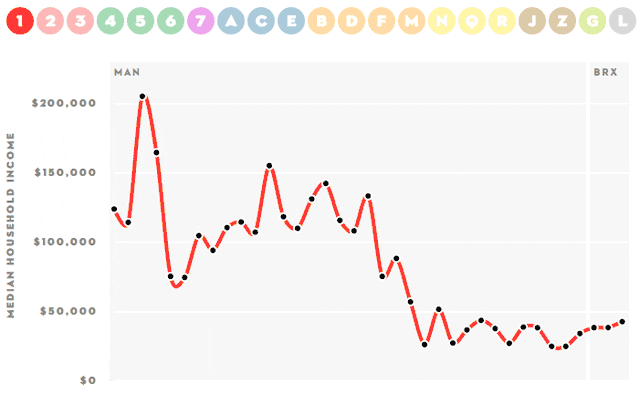## DEV Community# Data Visualization Basic Libraries

One of the advantages of using Python is user-friendly visualization packages. In this blog, I try to explain some essential plots for data visualization and I will share with you which plots I used for what before and what I found useful. Mainly, the plots which I will share in this article belongs to Matplotlib which is a library for Python using for visualization. Also, I would like to state about Seaborn, visualization library based on matplotlib, because I like Seaborn's colorful visuals. It is all about my usage preferences from my experiences.### Scatter Plot (plt.scatter)

First of all, we need to import matplotlib.pyplot like all other libraries, to use these plot types.

Scatter plots are very useful to understand the correlation between two variables easily. Also, I like to put markers for data points like 'stars','triangles' or 'sized-circles' according to the frequency of data. The most important feature which I like to use is scatter plot with a legend.Another feature that I like about scatter plot is adding a color bar with plt.colorbar(). It is very useful to see the colors and recognize different points. In the below graph, I used the 'viridis' as perceptually uniform sequential colormap scale but there are lots of alternative sequential, diverging, qualitative, miscellaneous color bars in 'matplotlib'.All of them can be found in matplotlib.org.

### Bar Plot (plt.bar)

I prefer bar plots to compare categorical data and differences between them. It is super easy to see the lengths of rectangular bars which proportional to data. Bars can be sorted according to y-axis values and the variables from best to worst easily can be seen.I like to color the bars also and compare two variables in same the graph with the bar plot. With this method, we can compare lots of variables with each other proportionally. Additionally, it is also good to arrange the width of the bars.### Pie plot (plt.pie)

When I would like to see the percentages of a small number of variables in the same graph, I like to use pie plots.Also, nested pie-plot charts can be created for more complex data but I do not prefer complexity for interpreting data. Another good visual thing, which I like about the pie plot is we can separate one slice from the pie plot and show the importance of this part.

### Histogram and Density Plot (plt.hist)

Undoubtedly, one of the best ways to see the distribution of the data is histograms. It is super easy to see the normally distributed variables which are a very important concept for data scientists. So, histograms are very essential graphs for data scientists.Although it is a great way to begin understanding a single variable distribution, histograms can fail when we try to compare distributions of one variable across multiple categories. Because, when histograms get together in the same area, readability will be the problem. The best way to do this is to create a new histogram for each category. Also, a side-by-side histograms or stacked bars can be useful.

There is a good example in towardsdatascience.com with NYC flight data about when histograms fail and how to solve this by using side-by-side histogram or stacked bars.

When density plots are get together with the histograms of the distribution of data, the graph will be more useful also. I want to state here about Seaborn which is super easy to use and Seaborn can create a histogram and density curve on the same plot easily.

### Seaborn (sns.pairplot , sns.heatmap , sns.distplot, sns.barplot)

Seaborn is a really useful and colorful library in Python. Like 'matplotlib' , we need to import Seaborn also before using it.

The most useful basic plots in Seaborn are pair plot, heat map, distribution plot. I also added a bar plot here because I like to use Seaborn's easy colorful bar plot. You do not need to give specific colors so, it is easier than 'matplotlib'. The other thing which I like about Seaborn is using fewer syntax.There are many ready datasets in Seaborn. This is the other important thing which I like. As a data scientist candidate, it is very good to find ready data sets to play around visualizing them. In the graph below, I plotted the distribution and also density curves for ready data set 'iris' which can be downloaded as sns.load_dataset('iris') easily.Another important thing for modeling is the correlation between independent variables. To analyze this correlation, Seaborn's sns.pairplot and sns.heatmap are useful functions.From the data set 'auto-mpg.csv', I choose some columns for simplification and just put my data set in pairplot as sns.pairplot(df). It is a super fast, easy and useful way of Seaborn. Then with the graph above, I can observe the correlations, linearity and also distribution of variables. So, sns.pairplot is a very important feature of Python.

It is also the same for heatmap.Just a simple one-line code I can observe all correlations with target and multicollinearity between variables super easily.

Lastly, there are many ways to visualize data in Python and also in other programming languages. But, these topics are really basic, useful and also easy to use for beginners. In addition to this, these are the fastest ways to observe datasets. As seen in this blog post, visualization library or tool always depends on the data and what you want to obtain.

Cover image sourced by Colin Behrens from Pixabay and gif is from giphy.